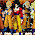## July 28, 2012

### Minimum vertex cover of tree

An unweighted, undirected tree is given. We have to find a vertex set of minimum size in this tree such that each edge has as least one of its end-points in that set, i.e. minimum vertex cover of tree.
The problem of finding a minimum vertex cover of a graph is a classical optimization problem in computer science and is a typical example of an NP-hard optimization problem. Taken as decision problem, it is NP complete. Tree is a special type of graph which has no cycles and so it's possible to find out the minimum vertex cover by using Dynamic Programming.
Basic idea is if any vertex is included in the set, we are free to choose or not choose it's children but if any vertex isn't included in set then we have to choose all the children of it.
In notation:
S+(u) = 1 + sum(min{S-(v), S+(v)})
S-(u) = sum(S+(v))

'u' is any node and 'v' refers to it's child[ren]
S is set which stores the vertexes of minimum cover. S+(u) means 'u' is present in set, S-(u) means 'u' is not present.
sum(X) means sum of all children

In my code I've taken root that vertex which is of highest degree. 'root' is the vertex from which query is started. After the whole tree is covered we take min{S+(root),S-(root)} as our answer. For pedant vertexes, S+(u)=1 and S-(u)=0 as it's trivial.

Original problem :
http://www.spoj.pl/problems/PT07X/

This is my solution

```#include <stdio.h>
#include <stdlib.h>
#include <string.h>

typedef struct node
{
// included, not included, neighbor
int inc, notinc;
int neigh;
}Node;

typedef struct vertex
{
int vertex_id;
struct vertex *next;
}Vertex;

#define MAX 100007

int n;
Node array[MAX];
Vertex *G[MAX]; // adjacency list representation of graph
short color[MAX]; // for visiting vetexes

inline int min (int P, int Q)
{
return (P<Q) ? P : Q;
}

void addNode (int u, int v)
{
Vertex *newvertex = (Vertex*)malloc(sizeof (Vertex));
newvertex->vertex_id = v;
newvertex->next = G[u];
array[u].neigh++;
G[u]=newvertex;
}
void dfs (int index)
{
if (array[index].neigh==1){
array[index].inc=1;
array[index].notinc=0;
color[index]=2; // visiting done
return;
}

int pos=0, neg=0;
color[index]=1;  // visiting in progress
Vertex *itr = G[index];
while (itr != NULL){
int curr = itr->vertex_id;
if (color[curr]==0){  // not visited yet
dfs(curr);
}
itr = itr->next;
}

itr = G[index];
while (itr != NULL){
int curr = itr->vertex_id;
if (color[curr] == 2){
pos += (min(array[curr].inc,array[curr].notinc));
neg += array[curr].inc;
}
itr = itr->next;
}
array[index].inc = pos+1;
array[index].notinc = neg;
color[index] = 2;
}

int main()
{
int I, a, b;
scanf("%d",&n);
memset (color, 0, sizeof color);

for(I=0;I<n+1;I++){
G[I]=NULL;
array[I].neigh=0;
}

for(I=1;I<=n-1;I++){
scanf("%d %d",&a, &b);
addNode(a, b);
addNode(b, a);
}
int root=1;
for (I=1;I<=n;I++){
if (array[I].neigh>array[root].neigh)
root = I;
}
if (n== 1 || n==2 || n==3)  //trivial cases. Handle on your own.
printf("%d",1);
else{
dfs (root);
printf("%d",min(array[root].inc, array[root].notinc));
}
return 0;
}
```

#### 1 comment:

1.which algorithm did you followed?